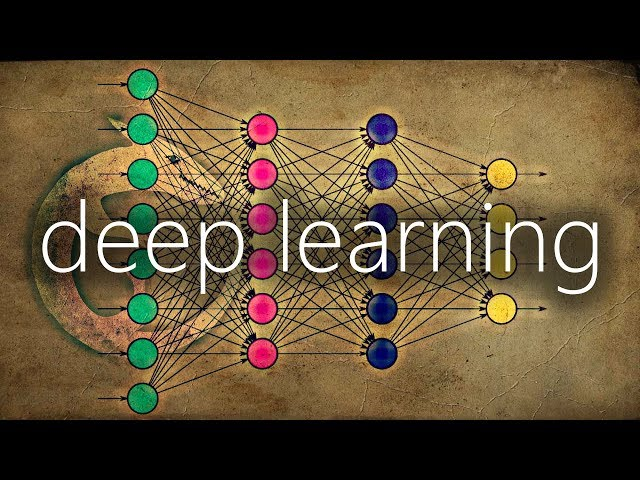# What is Cyclical Learning Rate

##### The Importance of Cyclical Learning Rates in Deep Learning

Deep learning has revolutionized the world of machine learning and has become an essential tool for data scientists, researchers, and engineers. However, deep learning can also be quite challenging due to its high complexity and the need for large amounts of data and computation power. One critical aspect of deep learning that can significantly impact its performance is the learning rate.

The learning rate determines the step size that the algorithm takes during the optimization process, affecting how quickly the model converges to a solution. If the learning rate is too low, the model may take a long time to converge, while if it is too high, the model may overshoot the optimal solution and diverge. Moreover, deep learning models often have a non-convex loss function, making it even more challenging to find the optimal solution.

Traditional learning rate schedules, such as a fixed learning rate or a learning rate that decreases over time, have been used for many years with some success. However, these methods can suffer from some limitations. For example, a fixed learning rate may be too high or too low, leading to suboptimal performance. On the other hand, a decreasing learning rate can become too small and slow down the optimization process. Therefore, researchers have been exploring new methods to improve the learning rate schedule, and one promising method that has emerged is cyclical learning rates.

Cyclical learning rates are a type of learning rate schedule that oscillate between two bounds during training, allowing the model to explore a more extensive range of learning rates and find a better solution. Typically, these bounds are set based on learning rate range test procedure, where the model is trained for a few epochs with a high learning rate, and the loss is monitored. The learning rate is then increased until the loss starts to diverge, giving us an upper bound, and then decreased again until the loss starts to stagnate, giving us a lower bound.

The idea behind cyclical learning rates is that they can help the model escape from local minima and saddle points, which are common in deep learning optimization. A local minimum is a point in the loss function where the model cannot improve anymore, and a saddle point is a point where the slope of the loss function is flat in some directions and steep in others, making it hard for the model to converge. Cyclical learning rates can help the model jump out of these points by oscillating between different learning rates, enabling it to explore different parts of the loss function.

Another advantage of cyclical learning rates is that they can also speed up the learning process by allowing the model to converge faster. Unlike traditional learning rate schedules, which may take many epochs to converge, cyclical learning rates can converge faster by making more significant adjustments to the learning rate, and therefore, the model parameters, in a shorter amount of time.

To implement cyclical learning rates in your deep learning models, you can use libraries such as PyTorch or TensorFlow, which provide built-in support for this technique. In PyTorch, you can use the "torch.optim.lr_scheduler.CyclicLR" class to define the cyclic learning rate policy, which takes as input the learning rate range, the cycling mode, and the step size.

• The learning rate range: This is the upper and lower bounds of the learning rate. Typically, these are set based on the learning rate range test procedure described earlier.
• The cycling mode: This determines how the learning rate oscillates between the upper and lower bounds. There are two cycling modes available: "triangular" and "triangular2." The triangular mode increases the learning rate linearly from the lower to the upper bound and then decreases it linearly back to the lower bound. The triangular2 mode is similar but uses a more complex triangular wave.
• The step size: This is the number of iterations (or epochs) that it takes for the learning rate to complete one cycle. This can be set based on the size of the dataset and the batch size.

Similarly, in TensorFlow, you can use the "tf.keras.callbacks.LearningRateScheduler" class to implement cyclic learning rates, which takes as input a function that takes the current epoch or iteration number and returns the appropriate learning rate. You can define any cyclic learning rate policy you like using this function, such as cosine annealing or a custom sinusoidal policy.

Finally, it's worth noting that there are several variations of cyclic learning rates, such as stochastic gradient descent with warm restarts (SGDR), which uses a cyclic learning rate schedule but also includes a "restart" phase where the learning rate is reset to the initial value and then cycles again. This can help the model explore different parts of the loss function more widely and can improve performance even further.

##### Conclusion

Cyclical learning rates are a useful technique for improving the performance of deep learning models by enabling them to escape local minima and saddle points and converge faster. By oscillating between different learning rates, the model can explore different parts of the loss function and find a better solution. If you're working on a deep learning project, you may want to consider using cyclic learning rates and experimenting with different variations of this technique to see how it affects performance.